# equivLiteral

## Overview

The equivLiteral method checks if the response from the student is literally equal to the correct value specified by the Author. This means that the form, order, elements, and values entered by the student should match the value in validation. Responses that are mathematically equal, but are in a form other than the one specified by Authors, will return false. Use equivLiteral when you want to strictly specify a particular form of the mathematical expression as the correct answer.

Note that equivLiteral ignores parentheses which have no specific meaning, by default.

• Allow decimal marks
Authors can specify what separators can be used by the student. From the Thousand separator drop-down menu, you can select dot, comma, and/or space. The Decimal Separator menu contains the option for either a dot or a comma. Note that the specified thousand separator and decimal separator cannot be the same, e.g. both dot.

• Ignore order
Ignores the order of expressions. E.g. both x+1 and 1+x will be considered correct. If this is not enabled, equivLiteral will not accept the correct response specified by the Author in a different order, even if it is an acceptable answer.

• Inverse result
Enabling this means that the value specified in the Value field will not be accepted as the correct answer. It is a useful way of excluding very specific answers from validation. Use case: Students are asked to enter something symbolically equivalent to `(x+2)^2`, however you don't want them to enter the same expression and score points for such response. In this case, adding an additional scoring method equivLiteral and enabling Inverse Result will exclude (x+2)^2 from correct response options. See examples in the Combining Methods section below.

• Allow interval
This option must be enabled when the students are expected to insert interval notation in the response area, ie  ‘[1, 4)’. Otherwise, the response will not validate correctly.
• Ignore trailing zeros
This option allows equivLiteral to ignore zeros after decimal separators in students responses. For example, when this option is enabled, 1000 and 1000.000 will be treated as equal.
• Ignore coefficient of 1
When enabled, the coefficient of 1 before a variable will be ignored. E.g. equivLiteral will treat 1x+2 and x+2 as equal.

• Treat 'e' as Euler's number
This option treats the variable 'e' as Euler's number. This overrides the default interpretation of 'e' as scientific notation.
• Compare Grouping
Enable this flag when requiring parenthesis grouping symbols with respect to the associative property of addition and multiplication. For example, when this option is enabled, x+(y+z) and x+y+z will not be treated as equal.

## Examples

Example 1 - Basic equivLiteral scoring

equivLiteral only accepts a response that is mathematically equal to the response set in the validation settings, and given in the same form. Note that parentheses that do not impact the mathematical result are ignored by default.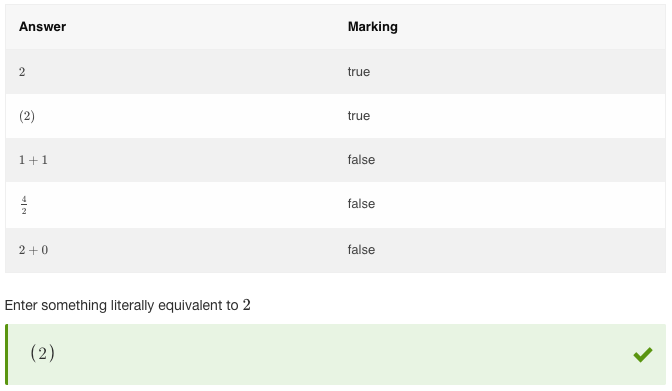Figure 1: Basic equivLiteral scoring

Example 2 - equivLiteral with decimals

When using equivLiteral with decimals, students will not be punished if they do not include leading zeros. However, the trailing zeros will be taken into account unless Ignore trailing zeros is enabled.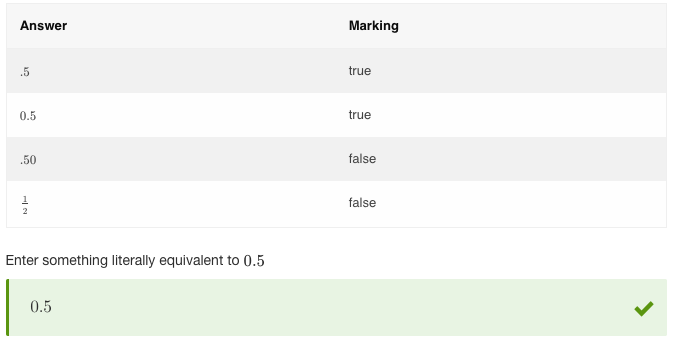Figure 2: equivLiteral with decimals

Example 3 - equivLiteral with decimals

This is the same question as above, only with the Ignore trailing zeros option enabled. This allows equivLiteral to ignore trailing zeros in students responses.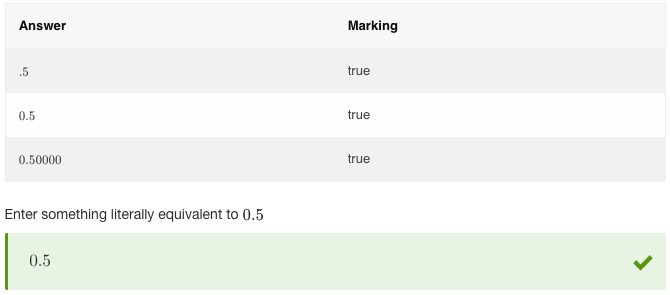Figure 3: equivLiteral with decimals

Example 4 - equivLiteral with fractions

When using equivLiteral with fractions, authors should bear in mind that only the fraction of the same form will be accepted. Decimal equivalent or other ways of expression will be considered incorrect.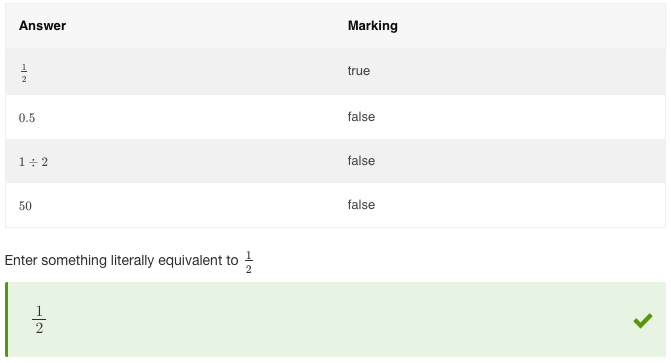Figure 4: equivLiteral with fractions

Example 5 - equivLiteral with variables

equivLiteral works with variables. In this example, the Ignore coefficient of 1 option is enabled. It allows equivLiteral to treat 1x and x as equal.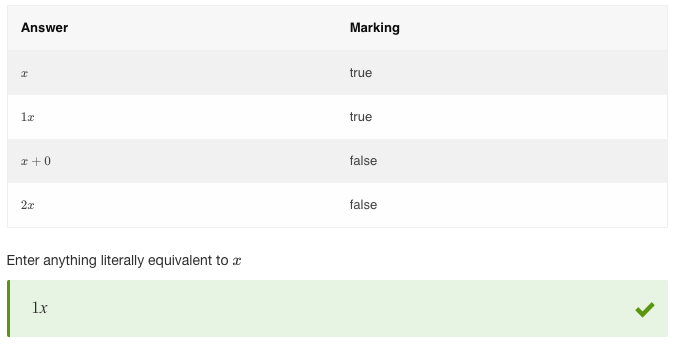Figure 5: equivLiteral with variables

## Combining Methods

Example - equivSymbolic + equivLiteral with Inverse Result

In this example, the flexible equivSymbolic scoring rules are constrained by a supporting equivLiteral method, with Inverse Result enabled. Valid responses in these two methods are the same, however putting it in the equivSymbolic settings allows correct responses in various formats, while equivLiteral ensures that students will not be able to score points for the exact same expression as the one in the question stimulus.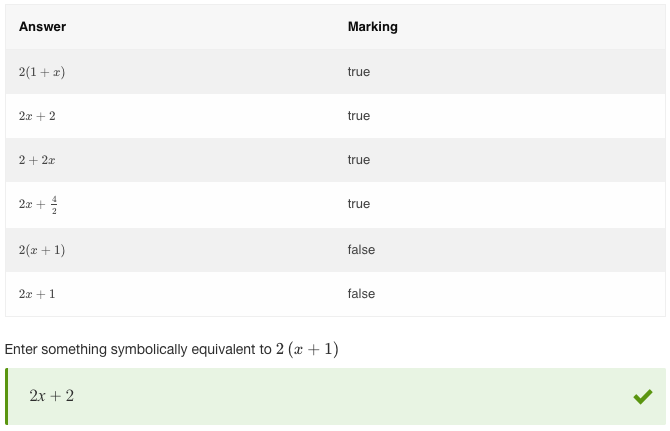Figure 6: Combining equivLiteral + equivSymbolic with Inverse Result